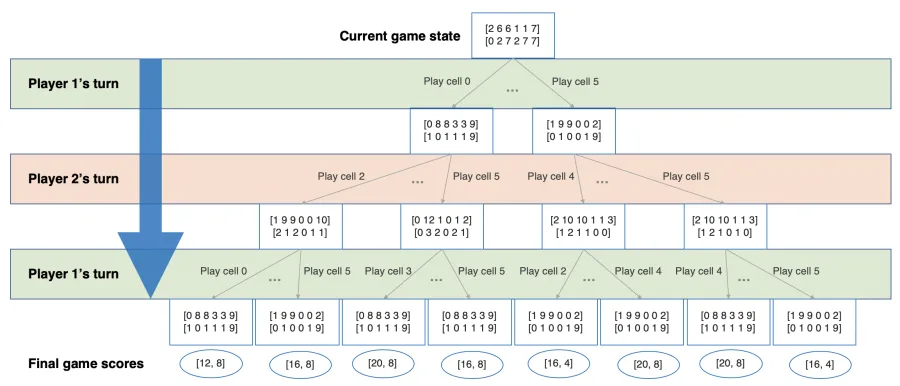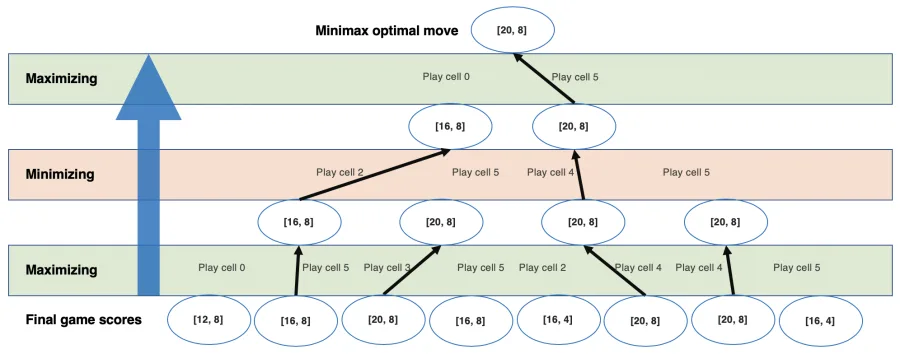Published on

# Building Ayòayò: An Unbeatable Player

Authors
•Name
Chidi Williams
@chidiwilliams__

In the previous posts in this series, we built a CLI and web game for Ayòayò (Ayò) with JavaScript. In this post, we'll implement an unbeatable AI player for the game using a decision rule called minimax.

## Types of games ​

Games have several different features or ways with which we classify them. We'll discuss two of these classifications: zero-sum vs non-zero-sum games and perfect information vs non-perfect information games.

A zero-sum game, like chess, is one in which players compete for a limited resource. A win for one player is a loss for the other(s). We say such a game is non-cooperative.

In a non-zero-sum (or positive-sum) game, however, actions are mutually beneficial (or mutually detrimental) to all players. Games like Minecraft and The Sims are cooperative non-zero-sum games.

Secondly, a game has perfect information if all players have complete knowledge of all the previous moves. Chess is also a perfect information game. At every point in a chess game, both players are aware of all the moves that led up to that point.

But in a non-perfect information game, like poker or rock-paper-scissors, players hold secret information that influences the outcomes of their opponent's moves.

In the case of rock-paper-scissors, because the moves happen simultaneously, it is impossible to know in advance what the outcome of your turn would be. You may be left thoroughly surprised as your opponent's paper covers your rock.

## The minimax strategy ​

In perfect information games, players act sequentially and can observe the state of the game before deciding what move to make. Each player acts to maximize the utility of their move.

We can represent such games with a decision tree that represents all possible game moves.

To choose the optimal strategy, we'll use a method known as backwards induction. Starting from the leaf nodes of the tree (which represent all the possible ways in which the game can end), we'll work our way up the tree, selecting the best node for each turn. The best node is the node that maximizes the utility of its player's move.

By the root node (the top of the tree), we would have chosen the best possible move the first player can make, while simultaneously assuming that their opponent is also making optimal moves.

If the game is also a zero-sum game, we can define the utility function as a single number which one player is trying to maximize and the other is trying to minimize. For example, we may define the utility function by asking: by how much is Player 2 winning? Player 1 will aim to minimize the value of the function while Player 2 aims to maximize its value.

This strategy of minimizing the potential loss (or maximizing the potential gain) is called minimax. The goal of minimax is to choose the optimal move while assuming the opponent is also playing optimally.

Minimax is well-suited for two-player zero-sum perfect-information games like Ayòayò.

## Minimax in Ayòayò ​

Let's consider an example to see how minimax works in Ayòayò.

We'll assume the game started with a board value of `[[2,6,6,1,1,7], [0,2,7,2,7,7]]` and that it is Player 1's turn to play next. What is the optimal move Player 1 can make to get the maximum benefit in the next three turns?

The following diagram shows the possible game moves for the next three turns and the final game scores. The diagram omits some moves for the sake of brevity (shown with "..."). We'll represent the game scores as `[player1Score, player2Score]`.The next diagram shows how we select the optimal minimax move. Moving upwards from the bottom of the tree, we select nodes according to whether the layer is maximizing or minimizing.

In the maximizing layers, we choose the child node whose value favours Player 1 the most. In the minimizing layers, we choose the child node whose value favours Player 2 the most.

The value at the top of the tree would be the minimax value: the optimal move to make assuming the opponent is also playing optimally.(Because minimax expects optimal opposition, it is usually possible for irrational real-life players to obtain higher-than-expected results by playing some sub-optimal moves.)

We'll now implement the minimax algorithm for Ayòayò step-by-step based on all the requirements of the algorithm.

### Traversing all possibilities ​

We'll start by building the decision tree of all the possible game moves.

js
``````function minimax(game, moves) {
if (game.winner != null) {
console.log(moves);
return;
}

game.permissibleMoves.forEach((move) => {
const gameCopy = game.clone();
gameCopy.play(move);
minimax(gameCopy, moves + move);
});
}

// Driver code
// minimax(new Ayoayo(), '')``````

The `minimax()` function recursively plays all possible game moves and prints out the moves.

However, the state space of the game is huge: there are 12 playable pits and 48 available stones. It would be impractical to traverse the entire game tree. We'll add a `depth` parameter to the function and stop the traversal after that number of turns.

Consequently, the algorithm would only consider the next `depth` moves and optimize for the final state at those moves.

js
``````function minimax(game, depth, moves) {
if (depth == 0 || game.winner != null) {
console.log(moves);
return;
}

game.permissibleMoves.forEach((move) => {
const gameCopy = game.clone();
gameCopy.play(move);
minimax(gameCopy, depth - 1, moves + move);
});
}

// Driver code
// minimax(new Ayoayo(), 3, '')``````

The runtime complexity for this traversal is `O(b^d)`, where `b` is the average branching factor of the tree and `d` is the depth we specify.

In each turn, we can play a maximum of 6 possible moves, so, the upper bound of the branching factor in Ayòayò is 6. However, as the game progresses, fewer pits are left to play. So the average branching factor would be a little less than 6.

We also see that the runtime increases exponentially with the specified depth.

### The utility function ​

Next, we'll define the utility function which the players are trying to minimize/maximize.

js
``game.captured - game.captured;``

Given this utility function, the goal of Player 1 would be to maximize this value while Player 2 aims to minimize this value.

(We could also set the score to `game.captured - game.captured`). In that case, Player 1 becomes the minimizer, and Player 2, the maximizer.)

### Minimizing (or maximizing) the utility function ​

For each branch node in the tree, we'll now attempt to minimize/maximize the value of its child nodes.

First, we'll add a `maximizing` boolean parameter to the function which specifies whether the player calling the function is trying to maximize or minimize.

js
``````function minimax(game, depth, moves, maximizing) {}

// Driver code
// minimax(new Ayoayo(), 3, '', true) // Maximizing. So, for Player 1.``````

For nodes in a maximizing level, we'll return the maximum score of its minimizing children. For nodes in a minimizing level, we'll return the minimum score of its maximizing children.

The full code for the minimax implementation becomes:

js
``````function minimax(game, depth, moves, maximizing) {
// Leaf node. Return the value of the utility
// function and the moves it took to get there.
if (depth == 0 || game.winner != null) {
return [game.captured - game.captured, moves];
}

if (maximizing) {
// Maximizing branch node. Return the maximum
// value of its minimizing children.
let maxScore = -Infinity;
let maxMoves;
game.permissibleMoves.forEach((move) => {
const gameCopy = game.clone();
gameCopy.play(move);
// Recurse into minimizing children.
const [score, childMoves] = minimax(gameCopy, depth - 1, moves + move, false);
if (score > maxScore) {
maxScore = score;
maxMoves = childMoves;
}
});
return [maxScore, maxMoves];
} else {
// Minimizing branch node. Return the maximum
// value of its maximizing children.
let minScore = +Infinity;
let minMoves;
game.permissibleMoves.forEach((move) => {
const gameCopy = game.clone();
gameCopy.play(move);
// Recurse into maximizing children.
const [score, childMoves] = minimax(gameCopy, depth - 1, moves + move, true);
if (score < minScore) {
minScore = score;
minMoves = childMoves;
}
});
return [minScore, minMoves];
}
}``````

(We can improve the performance of this algorithm with a technique known as alpha-beta pruning, but it's beyond the scope of this post.)

## Playing against minimax ​

Next, we'll add the minimax algorithm to the `Ayoayo` game. We'll write a function that creates a special instance of `Ayoayo` that plays the minimax move after each turn. In other words, it sets minimax as Player 2.

js
``````// Returns a new instance of Ayoayo that plays
// a minimax move after each call to play()
Ayoayo.vsMinimax = function vsMinimax(depth = 3) {
const game = new Ayoayo();
const oldPlayFunc = game.play.bind(game);
game.play = function minimaxPlay(...args) {
oldPlayFunc(...args);
if (game.winner == null) {
const [, moves] = minimax(game, depth, '', game.nextPlayer == 0);
const move = Number(moves);
oldPlayFunc(move);
}
};
return game;
};``````

Finally, we'll add a button to the web application to start a new game vs Minimax.

html
``<button class="new-game ai">New vs AI</button>``
js
``````const newAIGameButton = document.querySelector('.controls button.ai');
function onClickNewAIGame() {
game = Ayoayo.vsMinimax();
}``````

## Performance ​

Let's test how this algorithm performs. We'll play several games with one player playing the minimax move and the other playing a random allowed move.

We'll also use different depths for the minimax algorithm. An `n`-depth minimax player analyses `n` future moves starting from their next move: a 1-depth minimax player analyses only their next move; a 2-depth minimax player analyses their next move and the next move of their opponent; and so on.

Player 1Player 2Number of gamesPlayer 1Player 2Draw
1-depth MinimaxRandom25,00021,5561,1112,333
2-depth MinimaxRandom4,0002,66082258
3-depth MinimaxRandom500487013
Random1-depth Minimax25,00025022,9341,816
Random2-depth Minimax4,00083,90789
Random3-depth Minimax50004955
RandomRandom15,0004,5816,0954,324These results prove that increasing the depth of the constructed tree improves the success rate of the minimax algorithm (although it also increases the runtime exponentially as we've already seen).

We also learn from the minimax games and the Random vs Random game that the player with the first turn in a game of Ayòayò has a slightly lower chance of winning than the player who goes second.

Test your skills against the AI in the web game and check out the complete project for the series on GitHub.

A random fact about Ayòayò before you go: Yorùbá people have several different ways for greeting people on different occasions. During a game of Ayòayò in some Yorùbá communities, a spectator might say "Mo kí ọ̀tá, mo kí ọ̀pẹ́" meaning "I greet the champion and the loser". And another would respond "Ọ̀tá ni jẹ́, ọ̀pẹ́ ò lè f'ohùn" meaning "The champion responds, the loser can't talk".

Thanks for reading this post and the entire Ayòayò series!